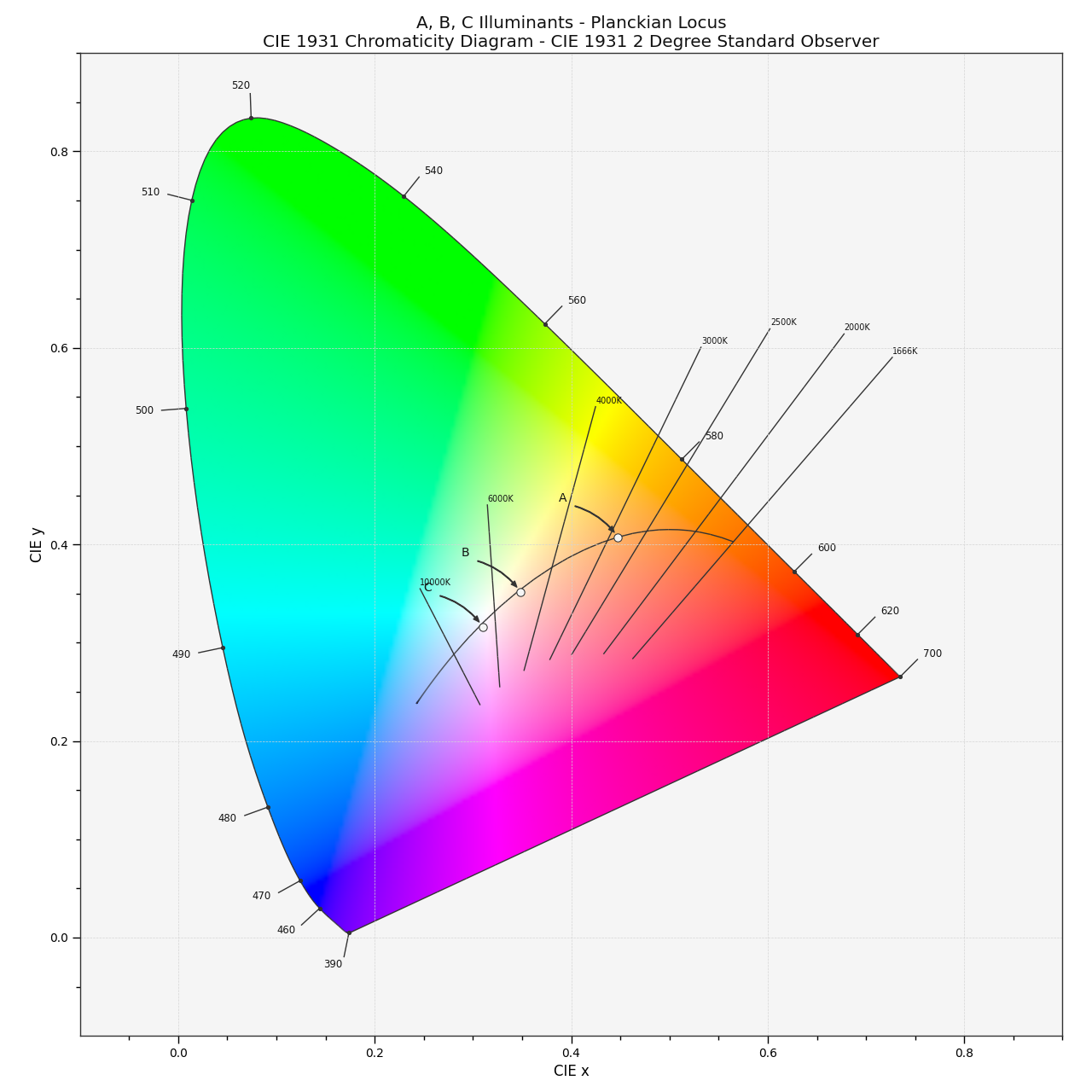# colour.plotting.plot_planckian_locus_in_chromaticity_diagram_CIE1931¶

colour.plotting.plot_planckian_locus_in_chromaticity_diagram_CIE1931(illuminants: Union[str, Sequence[str]], chromaticity_diagram_callable_CIE1931: Callable = plot_chromaticity_diagram_CIE1931, annotate_kwargs: Optional[Union[Dict, List[Dict]]] = None, plot_kwargs: Optional[Union[Dict, List[Dict]]] = None, **kwargs: Any) [source]

Plot the Planckian Locus and given illuminants in CIE 1931 Chromaticity Diagram.

Parameters
Returns

Current figure and axes.

Return type

`tuple`

Examples

```>>> plot_planckian_locus_in_chromaticity_diagram_CIE1931(['A', 'B', 'C'])
...
(<Figure size ... with 1 Axes>, <...AxesSubplot...>)
```